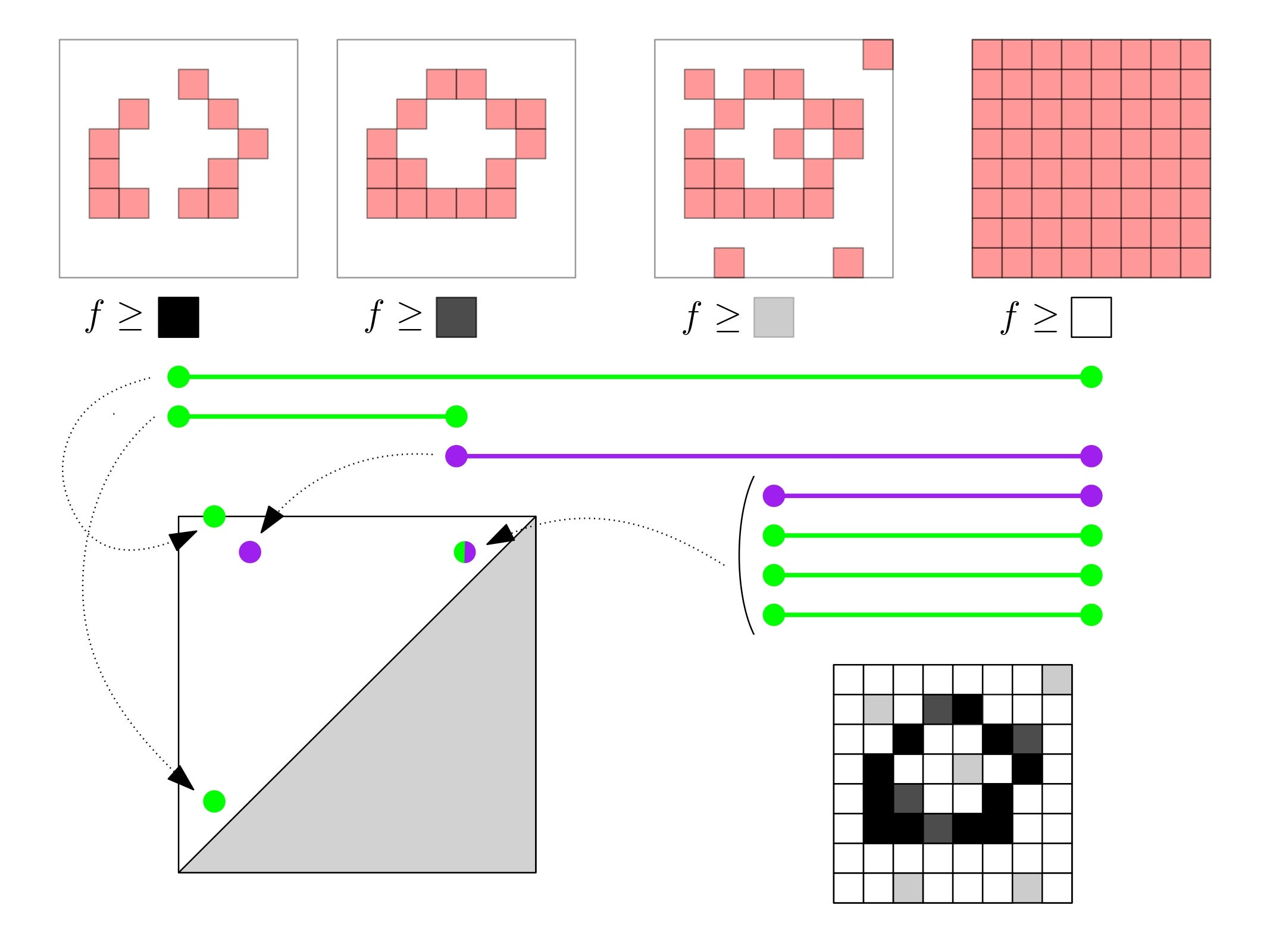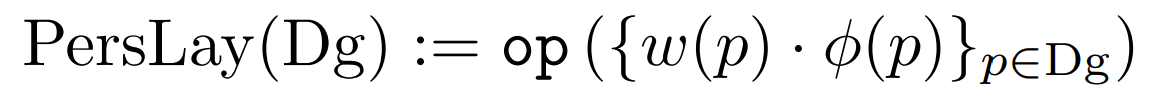# Mixing Topology and Deep Learning with PersLay

Deep LearningModelingPersLayTopologyposted by Mathieu Carrière March 2, 2020

In a former post, I presented Topological Data Analysis and its main descriptor, the so-called persistence diagram. In this post, I would like to show...In a former post, I presented Topological Data Analysis and its main descriptor, the so-called persistence diagram. In this post, I would like to show how these descriptors can be combined with neural networks, opening the way to applications based upon both deep learning and topology!

## What are persistence diagrams?

Briefly, a persistence diagram is a set of points in the plane that represents the topology of data: each point in this diagram actually witnesses the presence of a topological feature in the data. Such a feature can be a connected component (0-dimensional topology), a loop (1-dimensional topology), a cavity (2-dimensional topology) and so on.

In the example below, I show an example of a persistence diagram computed from the image of a zero (displayed in the lower right corner). The idea is to filter the image by progressively adding pixels. More specifically, we start with only those pixels whose grey value is more than a given threshold and we let this threshold decrease from large to low values until eventually, all pixels are present. In the process, several topological changes can happen (such as creation and merge of connected components and/or holes), and persistence diagrams exactly encode these changes. Each time a topological event happens, it gives rise to a point in the persistence diagram, whose coordinates provide the threshold values for which this event occurred.Example of computation of a persistence diagram from an image (source: https://www.dropbox.com/s/hihxt8cuz265bf9/phd.pdf?dl=0).

This descriptor is interesting especially because it encodes topological information, which is unusual, and very often complementary, to the information encoded by more traditional descriptors in machine learning. However, since the space of persistence diagrams is not structured (it is not possible to add two persistence diagrams in a consistent way for instance), several feature maps, i.e., embeddings of persistence diagrams into Hilbert spaces (such as finite-dimensional Euclidean spaces), have been defined over the past few years. Most of them are now available in a the scikit-learn compatible library sklearn_tda.

## PersLay: a layer for persistence diagrams

Today, I would like to introduce a handy tool that my coauthors and I have been developing during the past few months, which is called PersLay. PersLay is a neural network layer that is suited to handle persistence diagrams, and which generalizes most of the feature maps I mentioned above. More specifically, each feature map of the literature can be generated by PersLay with adequate parameters. This means in particular that the feature map can be learned on the fly during training time. No need for intensive cross-validation anymore!!

Moreover, since any subsequent neural network can be plugged after PersLay, it allows persistence diagrams to be treated by any architecture, whatever complicated it is.

The definition of PersLay is actually quite simple 🙂 Indeed, the primary requirement when one wishes to turn persistence diagrams into vectors in a differentiable way (so that it can be backpropagated) is to be invariant to permutations of the diagram points. Imagine you have to process two sets of points, whose only difference is in the point ordering: you probably want the outputs to be the same!

The simplest way to generate a permutation-invariant and differentiable feature map is probably to turn each point of the persistence diagram into a vector, and then sum over all such vectors to eventually get a single vector (actually, many other operations can be used instead of taking the sum, think about taking the maximum, the mean, the largest n values…). This is exactly the definition of PersLay.Definition of PersLay for a persistence diagram Dg. In this equation, w(p) is a weight function, phi is a vector transformation, and op is any permutation-invariant operation (source: https://arxiv.org/pdf/1904.09378.pdf).

## The modularity of PersLay

It turns out that all feature maps in the TDA literature actually fit into this general method! Depending on the way the diagram points are turned into vectors and on the permutation-invariant operation that is being used, one can show that applying the above method amounts to computing persistence imagespersistence landscapespersistence silhouettes

Take the persistence landscapes for instance. This method is one of the first feature maps that was ever defined to handle persistence diagrams. It is defined in the following way: for each point p of the plane, count the number of persistence diagram points for which this point p is located inside the square which has the persistence diagram point on its upper left corner. From this, you get a piecewise-constant function on the plane. The k-th persistence landscape is defined as the boundary of the area of the plane which contains all the points with function value k. This boundary is eventually turned into a vector of numbers by randomly sampling points and evaluating the persistence landscape on them.

Well, it turns out that persistence landscapes can be thought of as particular instances of PersLay! Indeed, an easier way to generate a persistence landscape is to associate a triangle function to each persistence diagram point and sample this function to get vectors. Then the k-th persistence landscape is nothing but the k-th largest value of each coordinate of these vectors!

## A simple architecture for persistence diagrams

This opens the use of persistence diagrams in a wide variety of tasks that was not accessible before! Indeed, even for thousands of persistence diagrams, it is way too expensive (in terms of running time) to cross-validate over all possible feature maps with traditional classifiers such as SVM or random forests. On the other hand, it can be done with a few lines of code with PersLay 😀

More precisely, the output of PersLay can be used as input for any subsequent neural network. In the article associated to PersLay, we study a simple architecture, in which several persistence diagrams are generated from each data point, and each of these diagrams is treated separately by a specific PersLay channel. The outputs of all channels are then concatenated (with some additional features), and we use a single fully-connected layer to produce a result with the right dimension.